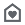Everyone
1,018

This app consists of a Gross Profit Calculator, Price Calculator, Operating Margin Calculator, Effective Interest Rate Calculator, Value Added Tax Calculator and a Break-Even Point Calculator.

Gross Profit Calculator: You are able to calculate the gross profit (absolute value and percentage) and markup (absolute value and percentage). Just enter the cost price and selling price of the product.

Price Calculator: You are able to calculate the price and markup. Just enter the cost and gross margin.

Operating Margin Calculator: You are able to calculate the operating margin. Just enter the operating income and revenue.

Effective Interest Rate Calculator: You are able to calculate the effective interest rate. Just enter the nominal interest rate and the number of compounding periods per year.

Value Added Tax: You are able to calculate the VAT (value added tax) and the price incl. VAT. Just enter the price without VAT and the VAT rate of your country.

Break-Even Point: You are able to calculate the break-even point. Just enter fixed cost, variable cost and price of a unit.
Collapse

## Reviews

Review Policy
4.1
1,018 total
5
4
3
2
1

Eligible for Family LibraryLearn More
Updated
April 18, 2016
Size
57k
Installs
100,000+
Current Version
2.7
Requires Android
1.6 and up
Content Rating
Everyone
Permissions
Offered By
GK Apps
Developer
Georg Kiefer Emser Str 75 56076 Koblenz Germany